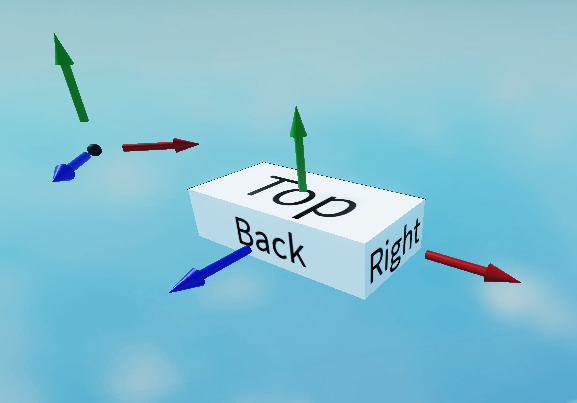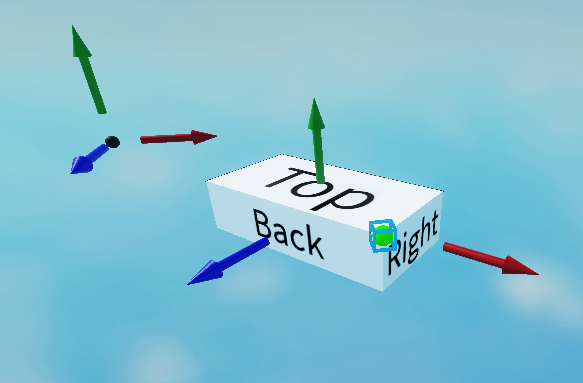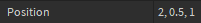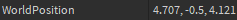# Comprehensive Beginner's Guide to CFrames and How to Use Them [CFrame Guide]

Here is a beginners guide to understanding and using CFrames. CFrames are super important and useful for any form of positioning and orienting of things in the workspace, so I hope this will help you with things like camera manipulation, even if you aren’t too good with the math involved.

Coordinate Frames

CFrame stands for ‘Coordinate Frame’, and this is a mathematical tool we use whenever we want to specify how something is positioned in space. A coordinate frame is made up of a point in space called the origin, and a set of 3 perpendicular directions called the axes (singular: ‘axis’). We usually name these 3 directions x, y, and z.(Here is a visual representation of a coordinate frame. In reality they are purely mathematical)

The `Position` property of a `Part` tells you its position according to the Workspace coordinate frame. By default, a part with a `Position` of `0,0,0` is positioned exactly at the origin of the `Workspace`. A part with a Position such as `3,5,-9` is displaced from the origin by 3 units along the x axis, 5 units along the y axis, and -9 units along the z axis (negative means backwards along the axis).

Along with the `Workspace`, some other types of instances also have their own associated coordinate frame, including `BasePart` and `Camera`. With `BasePart` specifically, its coordinate frame is attached so that its origin is located at the `Position` of the part, and its x, y, and z axes are facing the same direction as the part’s Right, Top, and Back sides respectively, always. What this means is that if you move the part in the workspace, you move the origin of it’s coordinate frame with it, and if you rotate the part, you rotate the axes of it’s coordinate frame. For this reason, the details of a part’s coordinate frame completely define the parts position and orientation in spaceThe `Orientation` property of a `Part` tells you how a part was rotated about the axes of its own coordinate frame. By default, a part with an `Orientation` of `0,0,0` has it’s Right, Top, and Back sides facing the same direction as the x, y, and z axes of the Workspace coordinate frame respectively. Equivalently, a part with that `Orientation` has the respective axes of its coordinate frame parallel with the axes of the `Workspace` coordinate frame. A part with an `Orientation` such as `45,90,30` is rotated along its y axis by 90 degrees, its x axis by 45 degrees, and its z axis by 30 degrees, in that order. Yes, order matters with rotation: if you did those same rotations in a different order, the faces (and the coordinate frame) would be oriented differently.

An important concept regarding coordinate frames is that the same point in space can have different positions depending on which coordinate frame you are using. This is because position is defined by displacement from the origin along the axes, and different coordinate frames have different origins and axes directions. A great example of this is an `Attachment`. The `Position` property of these is respect to the coordinate frame of it’s parent `Part`, instead of the `Workspace`.With respect to the `Part`:With respect to the `Workspace`:As of now, we have been talking about coordinate frames in the mathematical sense. But what is the meaning behind the actual `CFrame` property of an `Instance`? (I know, all this reading and we still haven’t talked about CFrames themselvesbut don’t worry, that was most of the learning: the rest is just understanding how to manipulate CFrames in code)

CFrame as a property of instances

Now that you know what a coordinate frame is, the purpose of a `CFrame` should be easily understood. `CFrame` is a property which encodes the position and orientation of a coordinate frame with respect to the workspace’s coordinate frame. This is why the `Workspace` doesn’t have a `CFrame` property, even though it has a coordinate frame: what’s the point of specifying your own position and orientation with respect to yourself?

If you have looked up CFrames before, you have probably seen that they are specified by 12 numbers. That may seem overwhelming at first, but its actually very simple: 3 numbers for the position of the origin of the coordinate frame, 3 for the direction vector of the x axis, 3 for the direction vector of the y axis, and 3 for the direction vector of the z axis (all with respect to the `Workspace` coordinate frame). That’s all. A `Part` positioned at the origin of the `Workspace` with the default orientation will have the 12 numbers of it’s `CFrame` consisting of `(0,0,0)`, `(1,0,0)`, `(0,1,0)`, `(0,0,1)`. That is to say, it’s coordinate frame’s origin is at the workspace origin, and its x, y, and z axes point parallel with the workspace’s x, y, and z axes respectively.

So why and how do you use CFrames? There are 2 main reasons you would want to use CFrames:

1. CFrames make positioning and orienting things simple and concise

This relates back to what I said earlier: the details of a part’s coordinate frame completely define the parts position and orientation in space (this goes for anything with a `CFrame`, including cameras). We can use this fact to easily code how we want things placed in the workspace. Using CFrames, along with their pre-defined functions that Roblox gives us, we can do things like both position and direct a `Camera` in only a couple lines of code:

(The camera is positioned at the origin, looking at the part.)

Here I used the `CFrame.lookAt(Vector3,Vector3)` constructor, which has the first argument being the `Position` of the CFrame, and the second argument being a point in space which the `LookVector` of the CFrame will point towards (the `LookVector` points opposite to the z axis of the coordinate frame, which is the direction of the Front face of a `Part` and the direction of view of a `Camera`)

In fact, it’s very rare that you will have to define a `CFrame` using 12 numbers. Most of the time you will use constructors like this one or manipulate pre-existing CFrames of other instances.

2. CFrames make it easy to position and orient things relative to other things

Let’s say I wanted I had one part called `part1` and I wanted it to be positioned 5 units on top of another part called `part2`. The problem is that the `Position` property of a part is with respect to the workspace, so we can’t just set it to `0,5,0`. This may be easy to fix with vectors if `part2` is nicely oriented in space: `part1.Position = part2.Position + Vector3.new(0,5,0)`, but what if `part2` is rotated beyond comprehension, and it’s top face isn’t nice enough to be directed along the y axis of the workspace (or any of the 3 axes for that matter)? Fear not, this is the mathematical purpose of coordinate frames after all. We know the position we want for `part1` in the coordinate frame of `part2`, we just need to convert that to the position in the workspace frame. We can use the handy function `CFrame:PointToWorldSpace(Vector3)`. This takes the position of a point in space specified with respect to the `CFrame`, and converts it to the position with respect to the workspace:
`part1.Position = part2.CFrame:PointToWorldSpace(Vector3.new(0,5,0))` does the trick for us.

Next I’ll talk a bit about some of the properties and particularly useful functions of CFrames and how to use them.

A quick rundown of some CFrame properties, functions and operations

This page lists everything + more that I’m about to talk about, but I’ll try go a bit more into detail than it does.

Properties:

• `CFrame.Position`: The `Position` of the coordinate frame’s origin
• `CFrame.LookVector`: The negative direction `Vector3` of the z axis of the coordinate frame. It’s named so because, as I said, it points in the direction of the Front face of parts, and the view of cameras
• `CFrame.UpVector`: The direction `Vector3` of the y axis of the coordinate frame.
• `CFrame.RightVector`: The direction `Vector3` of the x axis of the coordinate frame.

Operations:

• `CFrame + Vector3`: This returns the same `CFrame` with it’s `Position` shifted by the value of the `Vector3`. `CFrame - Vector3` behaves similarly
• `CFrame * Vector3`: Together, the direction `Vector3`'s of the x, y, and z axes of a coordinate frame actually form a 3x3 matrix which operates on `Vector3` values. This matrix can be thought of as instructions on how to rotate the axes of the workspace’s coordinate frame to become the axes of the `CFrame`'s associated coordinate frame. For this reason, this operation returns the original `Vector3` after it is rotated like the axes of the `CFrame` were. (For example, `Vector3.new(1,0,0))` will be rotated to point parallel to the x axis of the `CFrame`)
• `CFrame1 * CFrame2`: This takes both the the axes and position `Vector3` of `CFrame2` and rotates them according to the matrix of `CFrame1`. Additionally, this adds the `Position` of `CFrame1` to the previous result. The order matters here: you will get a different result if you swap `CFrame1` with `CFrame2`. If you you want a `CFrame` in the workspace which is positioned and oriented with respect to `CFrame1` as `CFrame2`, this will output it.

Check this out for a more in depth discussion on `CFrame` operations.

Functions:

• `CFrame1:Lerp(CFrame2, fraction)`: This takes the difference in `Position` and axis orientation of `CFrame1` and `CFrame2` and outputs one which is somewhere in the middle (specified by the fraction)
• `CFrame:Inverse()`: Returns a `CFrame` with the same `Position`, but it has the inverse matrix. This means if you had `vec2 = cf * vec1`, you would have `cf:Inverse() * vec2 == vec1`: it un-does the rotation of the original `CFrame`.
• `CFrame:PointToObjectSpace(Vector3)`: Similarly to `:PointToWorldSpace()` which I used in an example, this takes the position of a point according to workspace coordinate frame, and gives you it’s position according to the `CFrame` coordinate frame. You can think of `:PointToWorldSpace()` and `:PointToObjectSpace()` as being inverses of eachother.

Constructors:

• `CFrame.new(x,y,z)`: Creates a `CFrame` with a `Position` of `x,y,z`, and it’s axes parallel with the workspace’s (no rotation).
• `CFrame.Angles(X,Y,Z)`: Creates a `CFrame` positioned at `0,0,0` and rotate it about it’s own z, y, x axes by Z, Y, and X radians respectively, in that order. Remember that: Where as the `Orientation` property of parts uses degrees and goes in y,x,z order, this uses radians and goes z,y,x. You can use this constructor to make rotation matrices to transform `Vector3`s and other `CFrame`s
• `CFrame.lookAt(Pos, Target)`: As explained earlier, this creates a `CFrame` which is positioned at `Pos`, and points it’s `LookVector` at `Target`

I hope this guide wasn’t too long or confusing, but I also hope that it was in depth enough so that you came out with enough of an understanding of CFrames that you had the tools to do most of the things you would need them for, and so that you can look at the API page without the confusion about what any of it means.

Let me know if you have any suggestions or questions. Thanks for reading44 Likes

Absolutely outstanding tutorial! This explains CFrames very well, and you did a great job at explaining this! Very in-depth and very well-written. Keep it up, you have done amazing.

6 Likes

I am completely new to CFrames! This helped a lot! Thanks! Keep up the great work!

4 Likes

I finally pushed myself to start learning LUA and this is a great tutorial! I personally still am reading through, but its really well formatted!

That can help me a lot thanks1 Like

thank you for this help it will be greatly useful to me continue like this.1 Like